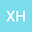The normalized Laplacian, degree-Kirchhoff index and spanning trees of graphs derived from the strong prism of linear hexagonal chain
•• Xiaocong He
Xiaocong He
Central South University

Corresponding Author:[email protected]

Author Profile## Abstract

Let L_n be a linear hexagonal chain with n hexagons. Let L^2_n be the graph obtained by the strong prism of a linear hexagonal chain with n hexagons, i.e. the strong product of L_n and K_2. In this paper, explicit expressions for degree-Kirchhoff index and number of spanning trees of L^2_n are determined, respectively. Furthermore, it is interesting to find that the degree-Kirchhoff index of L^2_n is almost one eighth of its Gutman index.